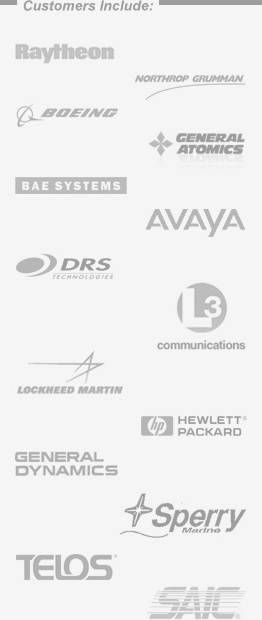Glossary

### V      [ABCDEFGHIJKLMNOPQRSTUVWXYZ]

VA - Volt-Amperes, product of volts times amperes, vectorial sum of Watts and VARs. Voltage (V) multiplied by the current (ampere); apparent power. For instance, a device rated at 10 amps and 120 V has a VA rating of 1200 or 1.2 kVA.

VAC - Voltage Alternating Current. The voltage measurement in an AC system.

VAR - Volt-Ampere Reactive, the reactive component of the VA.

VDC - Voltage Direct Current. The voltage measurement in an DC system.

VDE - A European safety agency that sets standards for product safety. See CE, UL, ETL, CSA and TÜV.

Voltage (volt or V) - The International System of Units measure of the potential difference between two electrical points, i.e. between line and neutral conductors. Voltage is the electrical pressure which forces the current to flow in a conductor such as a wire.

### W      [ABCDEFGHIJKLMNOPQRSTUVWXYZ]

WAN - Wide Area Network. A network which encompasses interconnectivity between devices over a wide geographic area.

Watt (W) - The unit of power in the International System of Units equivalent to 1 joule per second. The unit of measure for true power. Watts = VA x Power Factor

Watt-hour (Wh) - Electrical energy equivalent to 1 watt acting for 1 hour. This is also equivalent to 3.4136 BTUs (1 watt-hour = 1 joule/second x 3600 seconds/hour x 1 BTU/1054.6 joules = 3.416 BTUs).

WYE (Y) Connection  - A three-phase source of load connection, with a single common junction and three phase lines out or in.

### Z      [ABCDEFGHIJKLMNOPQRSTUVWXYZ]

Zero Voltage Switching (Zero Crossing Switching) - Technique in which the power switch in a converter turns on and off when there is a zero voltage across it. This minimized switching transient noise output from the converter.

*(denotes required field)
Contact ETI by mail at:
Energy Technologies, Inc.
219 Park Avenue East
Mansfield, OH 44902-1845
USA

Contact ETI by phone or fax:
Voice: 419-522-4444
FAX: 419-522-4466